Name:    1MD2

Multiple Choice
Identify the choice that best completes the statement or answers the question.

1.

How many inches long is the oval in the picture?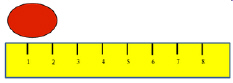a. 1 inch c. 3 inches b. 1/2 inch d. 2 inches

2.

How many inches long is the oval in the picture?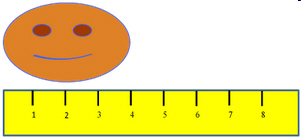a. 1 inch c. 3 inches b. 4 inches d. 2 inches

3.

How many inches long is the pink smiley face in the picture?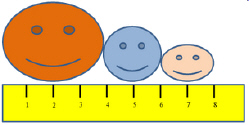a. 1 inch c. 8 inches b. 4 inches d. 2 inches

4.

How many inches long is the blue smiley face in the picture?a. 2 inches c. 8 inches b. 4 inches d. 1 inch

5.

How many inches long is the blue cloud in the picture?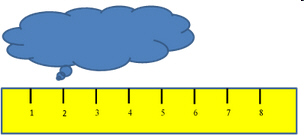a. 2 inches c. 6 inches b. 4 inches d. 5 inches

6.

How many inches long are all the smiley faces altogether?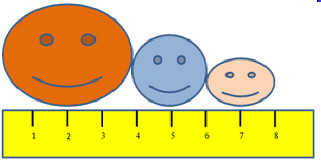a. 2 inches c. 7 inches b. 8 inches d. 1 inch

7.

How many inches long is the block in the picture?a. 5 inches c. 6 inches b. 1 inch d. 8 inches

8.

How many inches long is the triangle in the picture?a. 6 inches c. 7 inches b. 1 inch d. 8 inches

9.

How many inches long are the shapes in the picture altogether?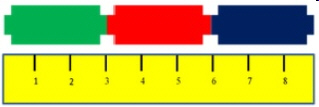a. 6 inches c. 7 inches b. 8 inches d. 9 inches

10.

How many inches long is the orange smiley face?a. 8 inches c. 3 inches b. 6 inches d. 4 inches